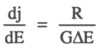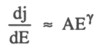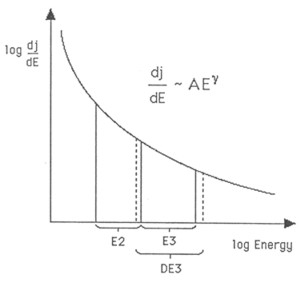ULYSSES

Ulysses HISCALE Data Analysis Handbook

## Appendix 9  Geometric Factor Study for the Deflected and Unscattered Electrons of HISCALE (Buckley MS Thesis)

The Ulysses spacecraft has five apertures, and all of these count charged particles.  Both of the LEMS detectors count ions, for example.  One advantage of having multiple detectors is that now one has the means of cross-checking one detector with another to see if counting rates are consistent--at least for isotropic events.  But before a direct comparison of counts can be made, an energy adjustment should be made to one of the detectors so that both data sets are seeing the same energy passband. For example, the energy passbands for E3 and DE3 are:

 Channel E(keV) E(keV) E3 175 103 DE3 170 107

As one can see, the passbands are close, but not exactly the same.

In Section A9.2, it was argued that for most practical purposes, differential fluxes could be represented by:where R is the raw spacecraft count rate DE = EHI - ELO, and G is the geometric factor. Typical energy spectra in space plasmas tend to follow a power-law spectrum, and we cna approximate this spectrum bywhere A is a constant, and g is the power-law exponent. Note that the gamma is typically between -1 and -4 for most solar events. Figure A9-44 shows a typical spectrum for an electron event; included in the figure are the upper and lower limits of each passband.

Figure A9-44 Illustration of spectrum fitting